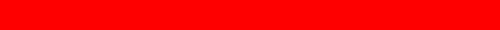# SUNDIAL

Back ] Up ] Next ]A

sundial is a simple instrument for reading time during daylight hours from the position of the Sun. However , comparison of sundial time, also called apparent time, with standard time usually shows a considerable difference, and the difference increases and decreases during the year. The rate of rotation of the Earth is remarkably uniform, varying only a tiny fraction of a second per year, but there are other factors in the Earth's motion that influence sundial time.

When placed on a standard meridian, the sundial will agree with a standard time clock on only four days of the year, running alternately ahead of and behind the standard clock. Since the standard clock is assumed to run at a uniform rate, the conclusion must be that the Sun does not move across the sky at the same rate throughout the year.

The standard clock keeps the time of an imaginary Sun, called the mean Sun, which is assumed to move uniformly throughout the year. Standard time is sometimes called mean time, referring to the mean Sun. The difference between the standard clock and the sundial is the same from year to year on the same date. The difference is tabulated as the equation of time. The equation of time is usually defined (and will be so used in this article) as:

EQUATION OF TIME = LOCAL APPARENT SOLAR TIME (sundial time) minus LOCAL MEAN SOLAR TIME (standard time).

Thus, if the sundial is ahead of the clock (reading later), the equation of time is positive, and if behind (reading earlier), it is negative. The equation of time is often displayed graphically on terrestrial globes as the narrow figure-8 curve, called the analemma.

 [ Back ] [ Up ] [ Next ]